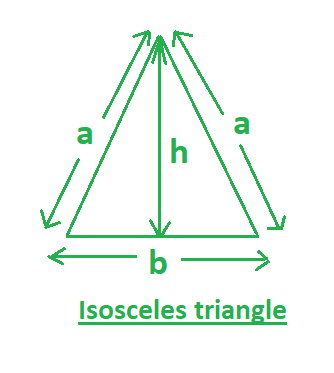# Find the altitude and area of an isosceles triangle

Given the side (a) of the isosceles triangle. The task is to find the area (A) and the altitude (h). An isosceles triangle is a triangle with 2 sides of equal length and 2 equal internal angles adjacent to each equal sides.In this figure,
a- Measure of the equal sides of an isosceles triangle.
b- Base of the isosceles triangle.
h- Altitude of the isosceles triangle.

Examples:

Input: a = 2, b = 3
Output: altitude = 1.32, area = 1.98

Input: a = 5, b = 6
Output: altitude = 4, area = 12


Formulas: Following are the formulas of the altitude and the area of an isosceles triangle.Below is the implementation using the above formulas:

## C++

 // CPP program to find the Altitude  // Area of an isosceles triangle  #include  using namespace std;     // function to find the atitude  float altitude(float a, float b)  {      // return altitude      return sqrt(pow(a, 2) - (pow(b, 2) / 4));  }     // function to find the area  float area(float b, float h)  {         // return area      return (1 * b * h) / 2;  }     // driver program  int main()  {         float a = 2, b = 3;      float h = altitude(a, b);      cout << setprecision(3);      cout << "Altitude= " << h << ", ";         cout << "Area= " << area(b, h);      return 0;  }

## Java

 // Java program to find the Altitude  // Area of an isosceles triangle     import java.io.*;     class GFG {               // function to find the atitude  static float altitude(float a, float b)  {      // return altitude      return (float)(Math.sqrt(Math.pow(a, 2) - (Math.pow(b, 2) / 4)));  }     // function to find the area   static float area(float b, float h)  {         // return area      return (1 * b * h) / 2;  }     // driver program            public static void main (String[] args) {              float a = 2, b = 3;      float h = altitude(a, b);      System.out.print( "Altitude= " + h + ", ");         System.out.print( "Area= " + area(b, h));      }  }  //This code is contributed by  inder_verma.

## Python 3

 # Python 3 program to find   # the Altitude Area of an  # isosceles triangle  import math     # function to find the atitude  def altitude(a, b):             # return altitude      return math.sqrt(pow(a, 2) -                      (pow(b, 2) / 4))     # function to find the area  def area(b, h):         # return area      return (1 * b * h) / 2    # Driver Code  if __name__ == "__main__":         a = 2     b = 3     h = altitude(a, b)      print("Altitude = " +             str(round(h, 3)), end = ", ")         print("Area = "+             str(round(area(b, h), 3)))     # This code is contributed   # by ChitraNayal

## C#

 // C# program to find the Altitude  // Area of an isosceles triangle  using System;     class GFG   {     // function to find the atitude  static float altitude(float a,                        float b)  {      // return altitude      return (float)(Math.Sqrt(Math.Pow(a, 2) -                              (Math.Pow(b, 2) / 4)));  }     // function to find the area  static float area(float b, float h)  {         // return area      return (1 * b * h) / 2;  }     // Driver Code  public static void Main ()  {      float a = 2, b = 3;      float h = altitude(a, b);      Console.WriteLine("Altitude = " + h + ", ");             Console.WriteLine("Area = " + area(b, h));  }  }     // This code is contributed   // by inder_verma

## PHP

 

Output:

Altitude= 1.32, Area= 1.98


Don’t stop now and take your learning to the next level. Learn all the important concepts of Data Structures and Algorithms with the help of the most trusted course: DSA Self Paced. Become industry ready at a student-friendly price.

My Personal Notes arrow_drop_upCheck out this Author's contributed articles.

If you like GeeksforGeeks and would like to contribute, you can also write an article using contribute.geeksforgeeks.org or mail your article to contribute@geeksforgeeks.org. See your article appearing on the GeeksforGeeks main page and help other Geeks.

Please Improve this article if you find anything incorrect by clicking on the "Improve Article" button below.

Improved By : inderDuMCA, vt_m, chitranayal